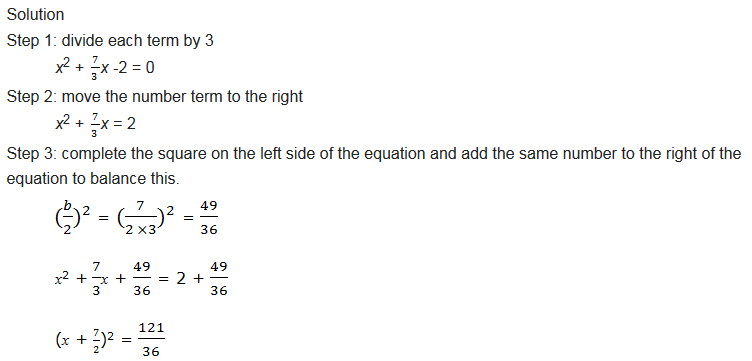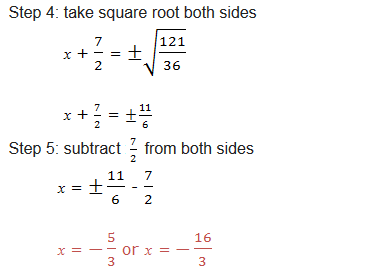# FORM TWO MATHEMATICS STUDY NOTES TOPIC 3-4

0
63Solving
quadratic equations can be difficult, but luckily there are several
different methods that we can use depending on what type of quadratic
that we are trying to solve. The four methods of solving a quadratic
equation are factoring, using the square roots, completing the square
The standard form of a Quadratic equation is ax2 + bx + c =0whereby a, b, c are known values and ‘a’ can’t be 0. ‘x’ is a variable (we don’t know it yet). a is the coefficient of x2 , b is the coefficient of x and c is a constant term. Quadratic equation is also called an equation of degree 2 (because of the 2 on x). There are several methods which are used to find the value of x. These methods are:
1. by Factorization
2. by completing the square
The Solution of a Quadratic Equation by Factorization
Determine the solution of a quadratic equation by factorization
We
can use any of the methods of factorization we learnt in previous
chapter. But for simplest we will factorize by splitting the middle
term. For Example: solve for x, x2 + 4x = 0
solution
Since the constant term is 0 we can take out x as a common factor.
So, x2 + 4x = x(x + 4) = 0. This means the product of x and (x + 4) is 0. Then, either x = 0 or x + 4 = 0. If x + 4 = 0 that is x = -4. Therefore the solution is x = 0 or x = -4.
Example 1
Solve the equation: 3x2 =- 6x – 3.
first rearrange the equation in its usual form.
that is:
3x2 = – 6x – 3
3x2 + 6x + 3 = 0
now, factorize the equation by splitting the middle term. Let us find two numbers whose product is 9
and their sum is 6. The numbers are 3 and 3. Hence the equation 3x2 + 6x + 3 = 0 can be written as:
3x2 + 3x + 3x + 3 = 0
3x(x + 1) + 3(x + 1) = 0
(3x + 3)(x + 1) (take out common factor which is (x + 1))
either (3x + 3) = 0 or (x + 1) = 0
therefore 3x = -3 or x = -1
x = -1 (divide by 3 both sides) or x = -1
Therefore, since the values of x are identical then x = -1.
Example 2
solve the equation 10 – 3y – 1 = 0 by factorization.
Solution
Two numbers whose product is -10 and their sum is -3 are 2 and -5.
Then, we can write the equation 10y2 – 3y – 1 = 0 as:
2y(5y + 1) – 1(5y + 1) = 0
(2y – 1)(5y + 1) = 0
Therefore, either 2y – 1 = 0 or 5y + 1 = 0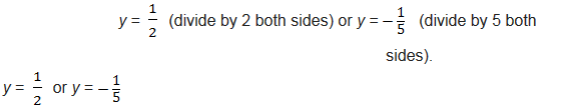Example 3
solve the following quadratic equation by factorization: 4x2 – 20x + 25 = 0.
Solution
We need to split the middle term by the two numbers whose product is 100 and their sum is -20. The numbers are -10 and -10.
The equation can be written as:
4x2 -10x – 10x + 25 = 0
2x(2x – 5) – 5(2x -5) = 0
(2x – 5)(2x – 5) (take out common factor. The resulting factors are identical. This is a perfect square)
since it is a perfect square, then we take one factor and equate it to 0. That is:
2x – 5 = 0
2x = 5 then, divide by 2 both sides.
Therefore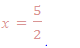Example 4
solve the equation x2 – 16 = 0.
Solution
We can write the equation as x2 – 42 = 0. This is a difference of two squares. The difference of two squares is an identity of the form:
a2 b2 = (ab)(a + b).
So, x2 – 42 = (x – 4)(x + 4) = 0
Now, either x– 4 = 0 or x + 4 = 0
Therefore x = 4 or x = -4
The Solution of a Quadratic Equation by Completing the Square
Find the solution of a quadratic equation by completing the square
Completing the square.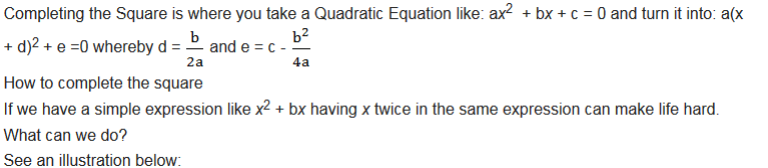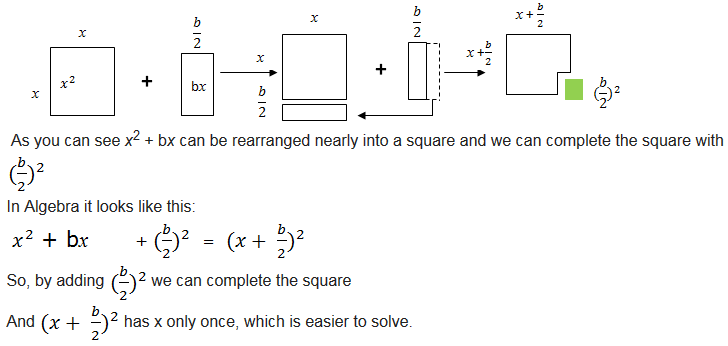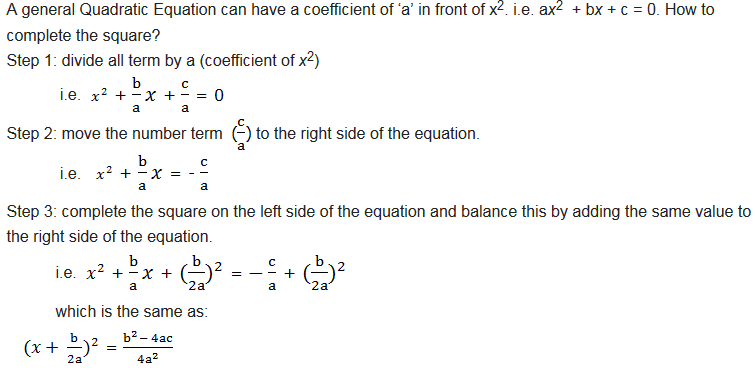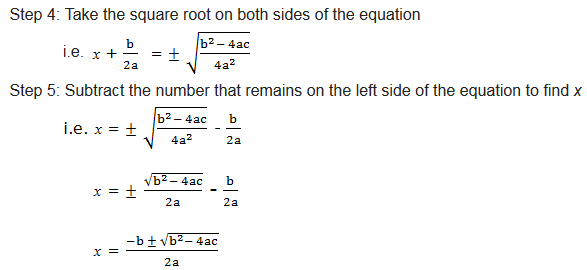Example 5
Add a term that will make the following expression a perfect square: x2 – 8x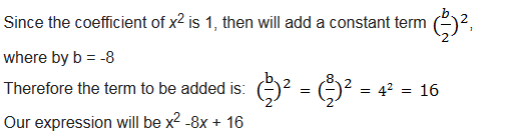find a term that must be added to make the following expression a perfect square: x2 + 10x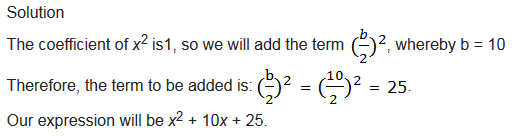Example 6
solve the following quadratic equation by completing the square: x2 + 4x + 1 = 0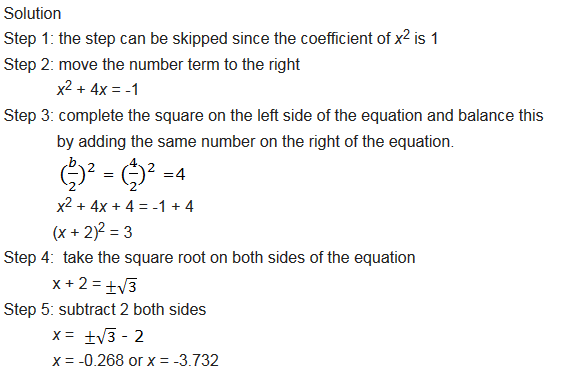Example 7
solve by completing the square: 3x2 + 7x – 6 = 0